# Sequence category

(diff) ← Older revision | Latest revision (diff) | Newer revision → (diff)

category of sequences

A particular case of the general construction of functor categories or diagram categories. Let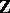be the set of integers equipped with the usual order relation. Thencan be considered as a small category with integers as objects and all possible pairs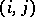, where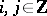and, as morphisms. The pairis the unique morphism from the objectto the object. Composition of morphisms is defined as follows: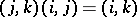.

For an arbitrary category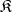, the category of functors fromtois called the category of sequences in. To define a functor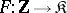, it is sufficient to indicate a family of objects from, indexed by the integers, and for each integerto choose a morphism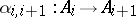. Then the assignment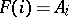,extends uniquely to a functor. A natural transformationfrom the functorto a functor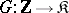, i.e. a morphism in the category of sequences, is defined by a family of morphisms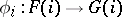such that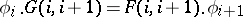for any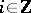.

Ifis a category with null morphisms, then in the category of sequences inone can isolate the full subcategory of complexes, i.e. functorssuch thatfor any. For any Abelian categorythe category of sequences and the subcategory of complexes are Abelian categories.

Instead of the categoryone can consider its subcategories of non-negative or non-positive numbers. The corresponding diagram categories are also called categories of sequences.